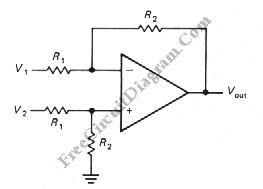# Differential Amplifier with Op-AmpThe schematic diagram below shows a differential amplifier circuit. This circuit is used to get a common-mode rejection ratios. This circuit need a precise resistor matching to get common-mode rejection ratios because the current source uses matched resistor ratios. Here is the circuit:The gain of this circuit is determined by following equation:
gain=R2/R1
The Vout is determined by following equation:
Vout=(R2/R1)*(V2-V1)
The best resistor of this circuit is 100k 0.01% resistors. This circuit has a unity gain, however using a further (single-ended) stages of gain the unity gain of this circuit remedied easily.